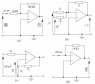# Opamps -- Unknown values

• j172
R/R*1+R/(R/2)*V_2 )So is there another step to this because this equation doesn't give V_2. do you transpose it ?It doesn't?Can you elaborate please

#### j172

Poster has been reminded to post schoolwork in the Homework Help forums (moved from EE)
Hi everybody!

This is my first post as I am needing some help in a question I am struggling with. Not looking for answers, just some guidance in the right direction.

## Homework Statement

[/B]
I need to calculate the unknown, highlighted, voltages on the op amp circuits (attached).

Assume all op-amps are ideal

See below

## The Attempt at a Solution

I have done the following so far:

a) Inverting
A=-Rf/Ri =(-100)/10=-10
Vi =I1*R1 =0.00001*100000=1 V
Vo=(-Rf/Ri )*Vi
Vo =1*-10
Vo =-10 V

b) Summing
Vo=-(Rf/R_1 V_1+Rf/R_2 V_2 )
-2=(Rf/R_1 1+Rf/(R/2) V_2 )

c) Non-inverting
V1 =V2
V1 =100 mV
V2 =100 mV

d) Current to Voltage
Vo=-I1*Rf
Vo =-0.0002*10000=-2
Vo =-2 V

I am struggling the (b). I can't see how to calculate given only one value, and also with (c)

Many thanks in advance, happy to discuss the methods.

Joel

#### Attachments

•Op Amps Question.png
16.6 KB · Views: 484
Last edited:
For A, Note that the voltage at both inputs to the op amp are equal, and at ground.
For B, I have 1R-2R+x(R/2)=0
For C & D, we agree.

j172 said:
a) Inverting
A=-Rf/Ri =(-100)/10=-10
Vi =I1*R1 =0.00001*100000=1 V
You have R1 wrong.
b) Summing
Vo=-(Rf/R V_1+Rf/R_2 V_2 )
-2=(Rf/R 1+Rf/(R/2) V_2 )
correct (I took out the "Rf" , should be just "R" everywhere)
c) Non-inverting
V1 =V2
V1 =100 mV
V2 =100 mV
Right.
d) Current to Voltage
Vo=-I1*Rf
Vo =-0.0002*10000=-2
Vo =-2 V
Right
I am struggling the (b). I can't see how to calculate given only one value, and also with (c)
The R's cancel. There is nothing more to do with (c) than you have already done.

•j172
Thank you very much for your help

b) Summing
Vo=-(R/R*V_1+R/R*V_2 )
-2=(R/R*1+R/(R/2)*V_2 )

So is there another step to this because this equation doesn't give V_2. do you transpose it ?

Last edited:
rob1985 said:
b) Summing
-2=(R/R*1+R/(R/2)*V_2 )
So is there another step to this because this equation doesn't give V_2. do you transpose it ?
It doesn't?

rob1985 said: